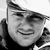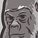# New to Qlik Sense

If you’re new to Qlik Sense, start with this Discussion Board and get up-to-speed quickly.

Announcements
Our next Qlik Insider session will cover new key capabilities. Join us August 11th REGISTER TODAY
cancel
Showing results for
Did you mean:Contributor II

## The column is missing totals

HI, i have problem. i use : if(Aggr(SUM(Document_PZ),Document_ZZ)=0,Sum([Document_ZZ]))
The column is missing totals. What can I do?

Sum(if(Aggr(SUM(Document_PZ),Document_ZZ)=0,Sum([Document_ZZ])) <---NOT WORK8 RepliesPartner

Your if statement is probably false for the total row.

SUM(Document_PZ),Document_ZZ)<>0

and therfore you gett null().

Try: sum(aggr( if(  SUM(Document_PZ)=0, Sum([Document_ZZ])),Document_ZZ))

I short, you need to create an expression that is valid for the expression without any dimension restrictions

Plees ekskuse my Swenglish and or Norweglish spelling misstakesContributor III

Hi

1. Edit Total by yourself.  Generally Total is placed in RowNo() = 0 or null.

If it is a Pivot Table, it is ColumnNo() = 0 or null

ex>  IF( RowNo() = 0 or Isnull(RowNo()), .....)

2. Use between two conditions "+"

ex>

if(Aggr(SUM(Document_PZ),Document_ZZ)=0,Sum([Document_ZZ]))  + if(Aggr(SUM(Document_PZ),Document_ZZ) <> 0, 0)Contributor II
Author

I gave the wrong data, they are correct:

if(Aggr(SUM(Quantity_Document_PZ),Document_ZZ)=0,Sum([Quantity_Document_ZZ]))Contributor II
Author

the ideas given above don't work.
maybe it's a quantity syntax problem?Contributor II
Author

have any ideas?MVP

You should include all dimensions which plot like

Sum(Aggr(if(Aggr(SUM(Document_PZ),Document_ZZ)=0,Sum([Document_ZZ])), Dimension1, Dimension2, etc.))

Before develop something, think If placed (The Right information | To the right people | At the Right time | In the Right place | With the Right context)MVP

If you wish to stick to If & Aggr I suggest following:

Sum( if(Aggr(nodistinct SUM(Quantity_Document_PZ),Document_ZZ)=0,[Quantity_Document_ZZ]))

or much better option which will be a lot better performing and not using Sum(If(Aggr: which gives me a satisfaction...

Sum({<Document_PZ={"=Sum(Quantity_Document_PZ)=0"}>}[Quantity_Document_ZZ])

hope this helps!

cheers Lech, When applicable please mark the correct/appropriate replies as "solution" (you can mark up to 3 "solutions". Please LIKE threads if the provided solution is helpful to the problem.Contributor II
Author

Thanks Lech

Sum({<Document_PZ={"=Sum(Quantity_Document_PZ)=0"}>}[Quantity_Document_ZZ])

this works!!!Tags
Community Browser